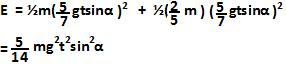## Wednesday, December 10, 2008

### Irodov Problem 1.252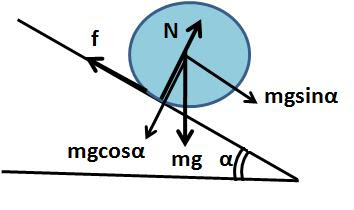There are three forces acting on the sphere, i) the force of gravity mg acting vertically downwards, ii) the normal reaction from the surface N and iii) the force of friction f that acts to resist any slipping between the sphere and the inclined plane acting in the direction of the slope of the plane. Let the acceleration of the sphere down the inclined plane be a.
In the direction of the slope of the inclined plane we have,In the direction perpendicular to the slope of the plane we have,Now let us consider the torques acting on the sphere. Since both normal reaction N and the force of gravity pass through the sphere's center of mass, they do not generate any torque on the sphere. The only torque that is responsible for the sphere's rotation is due to the force of friction given by fR. Let the angular acceleration of the sphere be. Since the moment of intertia of a sphere iswe have,If there is no slipping between the surface of the sphere and the inclined plane, then,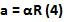From eqn (1), (3) and (4) we have,The maximum force of friction that any surface can provide is given by Nk, where k is the coefficient of friction. In order for no sliping to be there, the inclined surface must be able to provide the neccessary force of friction f to allow rolling given in (6). From (2) we have,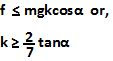b) If the velocity of the spehere is v and its angular velocity is, then the total kintetic energy of the sphere is the sum of i) translational kinetic energy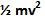and ii) the rotational kinetic energy given by.The velocity of the sphere as a fucntion of time is at and the angular velocity is at/R. So the total kinetic energy of the sphere will be,